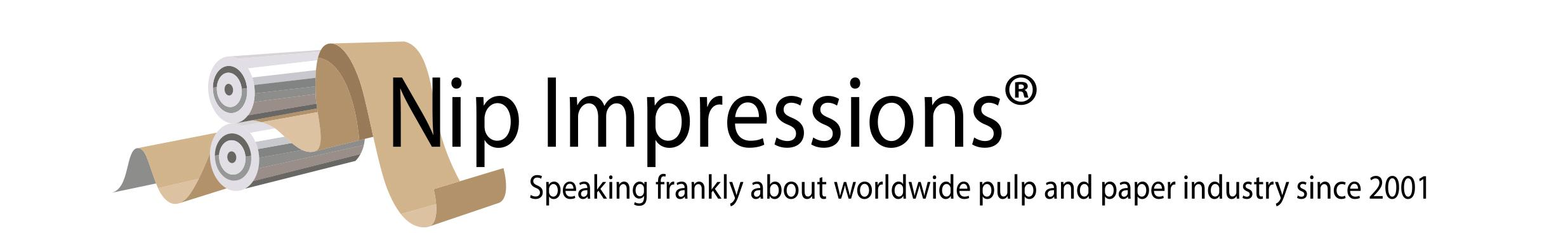Mon, May 16, 2022 21:25 Visitor

# Answer to this week's riddle

Again, the riddle:

Take a sheet of graph paper. Let's call a "Fred" a shape made up of contiguous squares that share at least one side. A "Fred" is original only if looks different than "Freds" of equivalent dimensions (the same number of squares) if flipped or rotated. Thus, there is 1 "Fred" of dimension 1 (a single square), 1 of dimension 2 (2 squares next to each other), 2 of dimension 3 (3 squares lying in a row or 3 squares in an "L"). How many "Freds" have dimension 4? How many have dimension 5?

A "Fred" is actually a polyomino, devised by Solomon Golomb in 1954. There are 5 tetrominoes (dimension 4 "Freds") and 12 pentominoes (dimension 5 "Freds"). See http://home.att.net/~numericana/answer
/gardner.htm#polyomino for more. (Also in "More" below)More...

Related Articles: